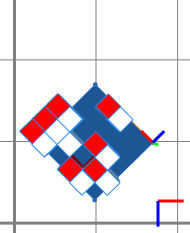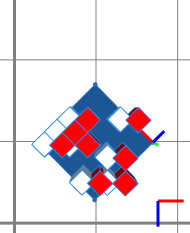# translate operation

### Syntax

• translate(mode, coordSystem, x, y, z)

### Parameters

1. modeselector
{ abs | rel }—Absolute or relative mode. Absolute means the position is set to the given value, relative means the translation is added.
2. coordSystemselector
{ scope | pivot | object | world }—Name of the coordinate system in which the following coordinates are given.
3. x,y,zfloat
The coordinates define a position in the coordSystem to which the current shape's scope (scope.t) is set if the mode is abs or a translation vector to apply if the mode is rel.

### Description

The translate operation translates the scope. The coordinates can be defined in any coordinate system, and the translation can either be absolute (= set to x,y,z) or relative (= add the x,y,z vector). This operation manipulates the scope position (scope.t attribute).

### Examples

#### Translate a shape along the world x-axis

 The red cubes are copies of the white cubes, translated by two units along the x-axis of the world coordinate system (i.e. the red axis on the bottom right). ``````Init --> split(x) { '0.2 : split(z) { '0.2 : PP }* }* PP --> 43% : primitiveCube() X translate(rel, world, 2, 0, 0) color("#ff0000") X else : NIL``````#### Translate a shape along the object x-axis

 The red cubes are copies of the white cubes, translated by two units along the x-axis of the object coordinate system (i.e. the red axis in the center). ``````Init --> split(x) { '0.2 : split(z) { '0.2 : PP }* }* PP --> 43% : primitiveCube() X translate(rel, object, 2, 0, 0) color("#ff0000") X else : NIL``````